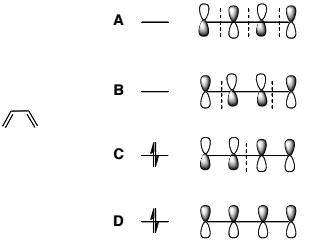# Problem: Consider the Molecular Orbitals (MO's) of butadiene. Which are the HOMO and LUMO? 1) HOMO = B, LUMO = C 2) HOMO = D, LUMO = A 3) HOMO = C, LUMO = B 4) HOMO = A, LUMO = D 5) HOMO = D, LUMO = B

🤓 Based on our data, we think this question is relevant for Professor Carrico's class at SUNY.

###### Problem Details

Consider the Molecular Orbitals (MO's) of butadiene. Which are the HOMO and LUMO?

1) HOMO = B, LUMO = C

2) HOMO = D, LUMO = A

3) HOMO = C, LUMO = B

4) HOMO = A, LUMO = D

5) HOMO = D, LUMO = B